# Shapes and Angles

## Objective

Measure angles that are greater than 180°.

## Common Core Standards

### Core Standards

?

• 4.MD.C.5 — Recognize angles as geometric shapes that are formed wherever two rays share a common endpoint, and understand concepts of angle measurement:

• 4.MD.C.6 — Measure angles in whole-number degrees using a protractor. Sketch angles of specified measure.

## Criteria for Success

?

Measure angles that are greater than 180° by:

1. Using a 360° protractor, if available (MP.5);
2. Understanding that the interior and exterior angles composed of three points must add up to 360°. Thus, finding the angle measure of the smaller angle will allow one to find the angle measure of the larger one by subtracting the smaller angle’s measure from 360° (MP.2, MP.3, MP.5); or
3. Breaking the large angle into two pieces, one of which is a straight angle. Since straight angles are 180°, one must only measure the non-straight angle of the pair and add its measure to 180° to find the angle measure of the large angle (MP.2, MP.3, MP.5).

## Tips for Teachers

?

Depending on the type of protractor students use to measure angles today, you may decide to move the location of this lesson. If students are using a 360° protractor, the lesson can be taught at this point in the unit (or even combined with Lesson 7, depending on block lengths and/or student readiness). If students are using a 180° protractor, you may decide to move this lesson until after Lesson 11, when students see that angles are additive, since the strategies for measuring an angle that is larger than 180° with a 180° protractor rely on this idea.

### Lesson Materials

• 180° Protractor (1 per student)

### Remote Learning Guidance

If you need to adapt or shorten this lesson for remote learning, we suggest prioritizing Anchor Task 1 (benefits from worked example). Find more guidance on adapting our math curriculum for remote learning here.

#### Fishtank Plus

• Problem Set
• Student Handout Editor
• Vocabulary Package

?

### Problem 1

Estimate the measure of $\angle QRS$.  Then, find the actual measure of $\angle QRS$ using a protractor.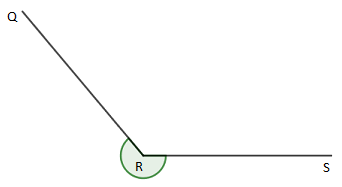#### References

EngageNY Mathematics Grade 4 Mathematics > Module 4 > Topic B > Lesson 7Concept Development Problem 2 and 3

Grade 4 Mathematics > Module 4 > Topic B > Lesson 7 of the New York State Common Core Mathematics Curriculum from EngageNY and Great Minds. © 2015 Great Minds. Licensed by EngageNY of the New York State Education Department under the CC BY-NC-SA 3.0 US license. Accessed Dec. 2, 2016, 5:15 p.m..

Modified by Fishtank Learning, Inc.

### Problem 2

Measure each of the following angles.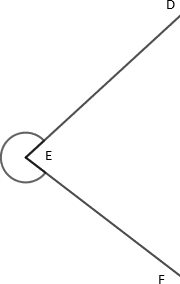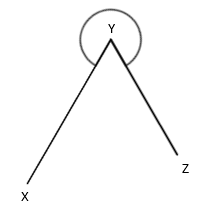## Problem Set & Homework

### Discussion of Problem Set

• It is important to learn to use the 180° protractor because it is the one you will see everywhere.  Explain to your partner how to measure an angle greater than 180° with a 180° protractor.
• Which strategy did you use the most for #1–8 and why did you choose that?
• What mistake did Maria make in #9?

?

Use a protractor to measure the following angles.

a.   Measurement: __________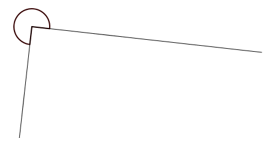b.   Measurement: __________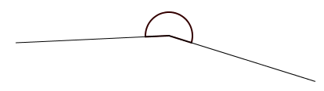c.   Measurement: __________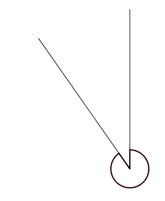d.   Measurement: __________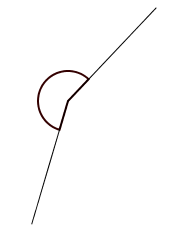#### Mastery Response